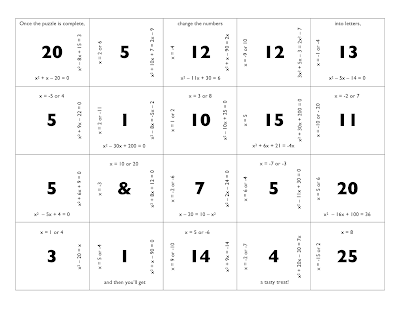## Tuesday, February 10, 2009

### Algebra 2: Factoring Trinomials (Part 1)Ok, so I guess it should really be titled Algebra 1, not 2. But my students always need to review/relearn this topic. We'll go easy for the first lesson - only problems where the GCF = 1 and where the leading coefficient is 1. I made a puzzle for them to put together so that it is more fun than just doing a worksheet. I did something like this in the past with my honors class (but with much harder polynomial equations) and they really enjoyed it. That puzzle, once assembled, instructed them to do push-ups to get some candy. This one only requires that they tell me a joke - I'll add them to my arsenal if they're any good.

Lesson 5 (Factoring Trinomials 1)
Puzzle (doc / pdf)

You may also be interested in the puzzle-based Treasure Hunt I did a couple years ago in Algebra 2.

## Saturday, February 07, 2009

### Algebra 2: Polynomials and FactoringWe just finished our first week of the second semester. The previous two weeks have been our Intersession period, where all students and teachers do totally different classes. This year, I did an algebra review class, helped organize our junior "boot camp" to help get them more ready for the college application process, and taught an anime class.

But now it's back to normal school, algebra 2, and time to start learning about polynomials. The first lesson was not that exciting, as we spent a lot of time learning all the needed vocabulary. But we also did learn about end behavior of polynomial functions, both graphically and algebraically. The next two lessons were more interesting, as we looked at the zero factor property from a graphical perspective, and then we learned how to sketch a polynomial function when given its linear factors graphically. This is scaffolding for the number line model lesson that will happen on Monday, which will allow students to solve factored form polynomial inequalities like (x - 3)(2x + 5) < 0. This isn't in the algebra 2 standards, but this kind of analysis will push them to understand functions more deeply, so I think it is worth the time.

In lesson 3, students worked as a class to generate sketches of product functions by multiplying the linear factors. They really caught on, and were able to easily get through the first problem pictured in this post. It was great to watch them work together as a class so well. The goal is that, on Monday, they will be able to understand and solve the second problem in this post.

Here are the files:
Lesson 1 (Classifying Polynomials / End Behavior) word / keynote / quicktime
Lesson 2 (Zero Factor Property) word / keynote / quicktime
Lesson 3 (Curve Sketching - Graphically) word / keynote / quicktime
Lesson 4 (Curve Sketching - Analytically) word / keynote / quicktime